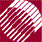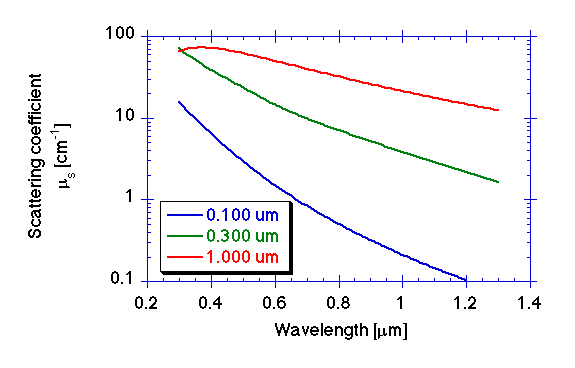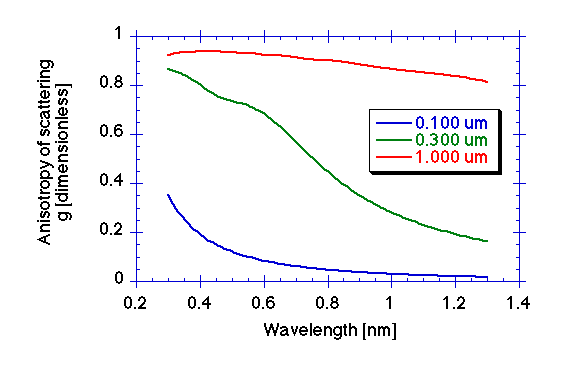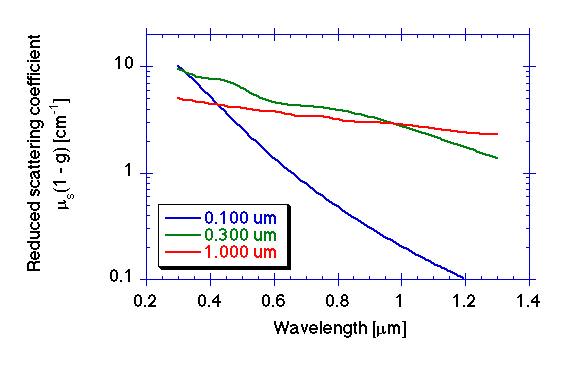ECE532 Biomedical Optics © 1998 Steven L. Jacques, Scott A. Prahl Oregon Graduate Institute

# Scattering versus wavelength for 3 particle sizes

Consider the three optical scattering properties, µs, g, and µs(1 - g), as functions of wavelength for a spherical particle with np = 1.5721 in a medium with nmed = 1.3316 at a concentration of 0.1% volume fraction (fv = 0.001). Assume that np and nmed are constant versus wavelength for this example calculation.

Let the sphere be one of three sizes: 0.100 µm, 0.300 µm, and 1.00 µm.

The Mie theory calculation yields:

## µs## g## µs(1 - g)Note: The true np and nmed for polysytrene spheres and water are slightly wavelength dependent and would deviate slightly from the above example.